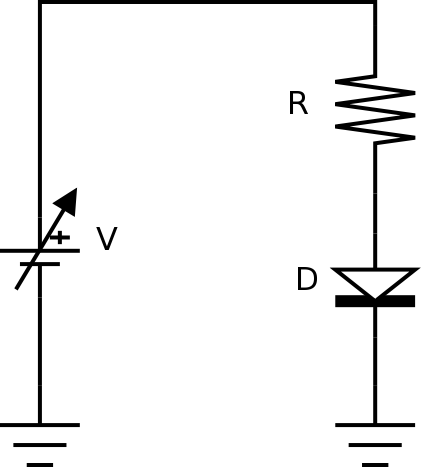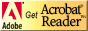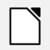# PC221 Analog Electronics I

## Diodes and DC Sweeps

### Objectives

1. To introduce the use of variable DC supplies.
2. To review the use of the function generator.
3. To introduce plots of IV curves.
4. To expand the use of SPICE circuit simulation to include DC sweeps.
5. To compare results of measurement, and simulation.

### Equipment

• digital multimeter, variable DC supply, oscilloscope
• resistor and diode

### Background

In this lab and the one that follows, you're not going to do Kirchhoff's analysis of the circuits, and you aren't going to use both the digital meter and the oscilloscope for all of the same measurements. Instead, circuit measurements can use whatever instrument is appropriate, and the comparisons will only be between the measurements and the simulation values.

Often you want to plot I-V curves for devices for circuits.

#### IV curves in SPICE

There are at least a couple of ways to plot IV curves using SPICE:

• Do a transient analysis, and plot voltage versus current instead of versus time.
• Do a DC sweep of the voltage, and plot voltage versus current.

In order to show you how it's done, you'll use the latter method in the lab. (DC sweeps can be done of many things, such as temperature or resistance values; not just voltages.)

### Procedure

#### Setup

1. Assemble the components for the following circuit:The values are
• V = variable DC supply, from -10V to +10V
• R = 1.0 kΩ
• D = 1N4004

#### Circuit Measurement: Digital Multimeter

2. Measure the resistance with the digital meter.

3. Read Checking Diodes, and test the diode in forward and reverse directions. Could you tell which direction was forward biased by measurements alone?
Demonstrate and explain diode checking to the lab instructor.

4. Build the circuit, and determine the diode current and voltage over the range stated above.

For the DC supply,
• to do negative voltages, reverse the supply polarity
• use the digital meter to measure the voltage across R
• use the digital meter to measure the voltage across D
• use the resistor voltage to determine the current through R (and D)

5. Make an I-V curve of the diode using your measurements.

#### Circuit Visualization: Oscilloscope

6. Replace the DC supply with a sine wave from -10 to +10 V (or as close as you can get to that) at a frequency of 1 kHz. Observe the diode voltage with the oscilloscope.
• Sketch the voltage across the diode for at least two cycles of the sine wave.
7. Summarize the operation of the diode, given what you know about diodes.
Explain your results to the lab instructor before continuing.

#### Circuit simulation: Introducing DC Sweep

8. If using LTspice:
SPICE can do many different types of circuit analysis.

Use the information in our LTspice tutorial to do a DC sweep.

The actual diode you'll be using isn't included in the LTspice libraries, so you'll have to use the information on adding a model in LTspice to add a model to the SPICE library. (There are three different methods shown; use whichever one works.) Method 3 may be the easiest.
You can find a SPICE model at ON Semiconductor. (Search for the part number and then look for information about models.)

If using CircuitLab:
If you have another diode in the 1N400x family, where x is a digit, you can use it but you'll need to find out what the difference is between the members of the 1N400x family, and whether that should affect what you need to do in this lab.

If using LTspice or CircuitLab:
Do a DC sweep, and from the DC sweep, plot the diode current versus the diode voltage to compare with the graph above.

9. Do a transient (or time domain) analysis to produce a graph of voltage across the diode versus time to compare with the sketch and the DC sweep from above.

#### Comparing measured and simulated values

10. Compare the values from the actual circuit to the values from your analysis and measurements above.
Show your results to the lab instructor.

11. Save your circuit and your LTspice files (or CircuitLab project) for future use.

Before you leave the lab, have the lab instructor sign your lab notebook immediately after your last entry.

## ResourcesIf you need to update a browser, you might try Firefox which is
• free
• open source
• available for several platformsSince this page uses cascading style sheets for its layout, it will look best with a browser which supports the specifications as fully as possible.

If you are looking for an office package, with a word processor, spreadsheet, etc., you might try LibreOffice which is
• free
• open source
• available for several platformsGo to the main page for the Department of Physics and Computer Science.

Wilfrid Laurier University